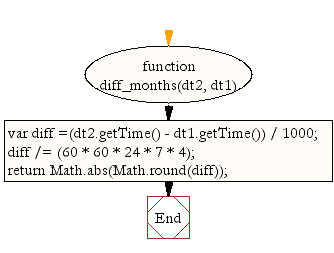# JavaScript: Get time differences in months between two dates

## JavaScript Datetime: Exercise-48 with Solution

Write a JavaScript function to get time differences in months between two dates.

Test Data:
dt1 = new Date("June 13, 2014 08:11:00");
dt2 = new Date("October 19, 2014 11:13:00");
console.log(diff_months(dt1, dt2));
5

Sample Solution:

HTML Code:

``````<!DOCTYPE html>
<html>
<head>
<meta charset="utf-8">
<title>JavaScript function to get time differences in months between two dates</title>
</head>
<body>

</body>
</html>
```
```

JavaScript Code:

``````function diff_months(dt2, dt1)
{

var diff =(dt2.getTime() - dt1.getTime()) / 1000;
diff /= (60 * 60 * 24 * 7 * 4);
return Math.abs(Math.round(diff));

}

dt1 = new Date(2014,10,2);
dt2 = new Date(2014,10,11);
console.log(diff_months(dt1, dt2));

dt1 = new Date("June 13, 2014 08:11:00");
dt2 = new Date("October 19, 2014 11:13:00");
console.log(diff_months(dt1, dt2));
```
```

Sample Output:

```0
5
```

Flowchart:Live Demo:

See the Pen JavaScript - Get time differences in months between two dates-date-ex-48 by w3resource (@w3resource) on CodePen.

Improve this sample solution and post your code through Disqus

What is the difficulty level of this exercise?

﻿

## JavaScript: Tips of the Day

Convert tabs to spaces, where each tab corresponds to count spaces

Example:

```const tips_expandTabs = (str, count) => str.replace(/\t/g, ' '.repeat(count));
console.log(tips_expandTabs('\t\tw3resource', 4));
```

Output:

```"        w3resource"
```